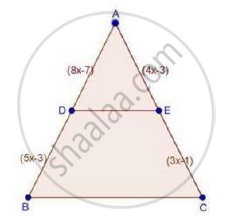Share

# In δAbc, D and E Are Points on the Sides Ab and Ac Respectively Such that De || Bc If Ad = X, Db = X − 2, Ae = X + 2 and Ec = X − 1, Find the Value of X. - CBSE Class 10 - Mathematics

ConceptBasic Proportionality Theorem Or Thales Theorem

#### Question

In ΔABC, D and E are points on the sides AB and AC respectively such that DE || BC

If AD = x, DB = x − 2, AE = x + 2 and EC = x − 1, find the value of x.

#### SolutionWe have,

DE || BC

Therefore, by basic proportionality theorem,

We have,

"AD"/"DB"="AE"/"EC"

rArrx/(x-2)=(x+2)/(x-1)

⇒ x(x − 1) = (x + 2)(x − 2)

⇒ x2 − x = x2 − (2)2    [∵ (a – b) (a + b) = a2 − b2]

⇒ −x = −4

⇒ x = 4 cm

∴ x = 4 cm

Is there an error in this question or solution?

#### Video TutorialsVIEW ALL 

Solution In δAbc, D and E Are Points on the Sides Ab and Ac Respectively Such that De || Bc If Ad = X, Db = X − 2, Ae = X + 2 and Ec = X − 1, Find the Value of X. Concept: Basic Proportionality Theorem Or Thales Theorem.
S